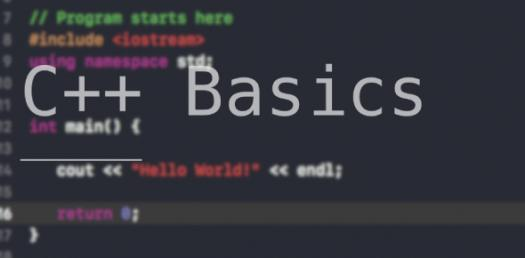# Basics Of C++ Trivia Questions Quiz

7 Questions | Total Attempts: 141SettingsC++ is a programming language that is widely used in developing a lot of applications such as gaming engines because of how fast it is. Are you about to sit for exams about the basics behind this language? The quiz below is exactly what you need to ensure you get the best marks. Do take up others like it.

• 1.
What is the correct value to return to the operating system upon the successful completion of a program?
• A.

-1

• B.

1

• C.

0

• D.

Programs do not return a value.

• 2.
What is the only function all C++ programs must contain?
• A.

Start()

• B.

System()

• C.

Main()

• D.

Program()

• 3.
What punctuation is used to signal the beginning and end of code blocks?
• A.

{}

• B.

->and

• C.

BEGIN and END

• D.

( and )

• 4.
What punctuation ends most lines of C++ code?
• A.

.

• B.

;

• C.

:

• D.

'

• 5.
Which of the following is a correct comment?
• A.

• B.

** Comment **

• C.

/* Comment */

• D.

{ Comment }

• 6.
Which of the following is not a correct variable type?
• A.

Float

• B.

Real

• C.

Int

• D.

Double

• 7.
Which of the following is the correct operator to compare two variables?
• A.

:=

• B.

=

• C.

Equal

• D.

==

Related TopicsBack to top